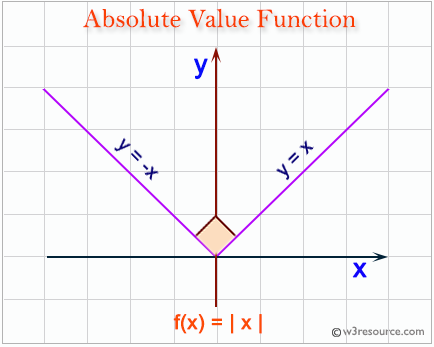# Python: Test whether a number is within 100 of 1000 or 2000

## Python Basic: Exercise-17 with Solution

Write a Python program to test whether a number is within 100 of 1000 or 2000.
Python abs(x) function:
The function returns the absolute value of a number. The argument may be an integer or a floating point number. If the argument is a complex number, its magnitude is returned.Sample Solution:-
def near_thousand(n):
return ((abs(1000 - n) <= 100) or (abs(2000 - n) <= 100))
print(near_thousand(1000))
print(near_thousand(900))
print(near_thousand(800))
print(near_thousand(2200))

============ RESTART: F:/Python_APSC/py-ex-basic-17.py ===========
True
True
False
False
>>>